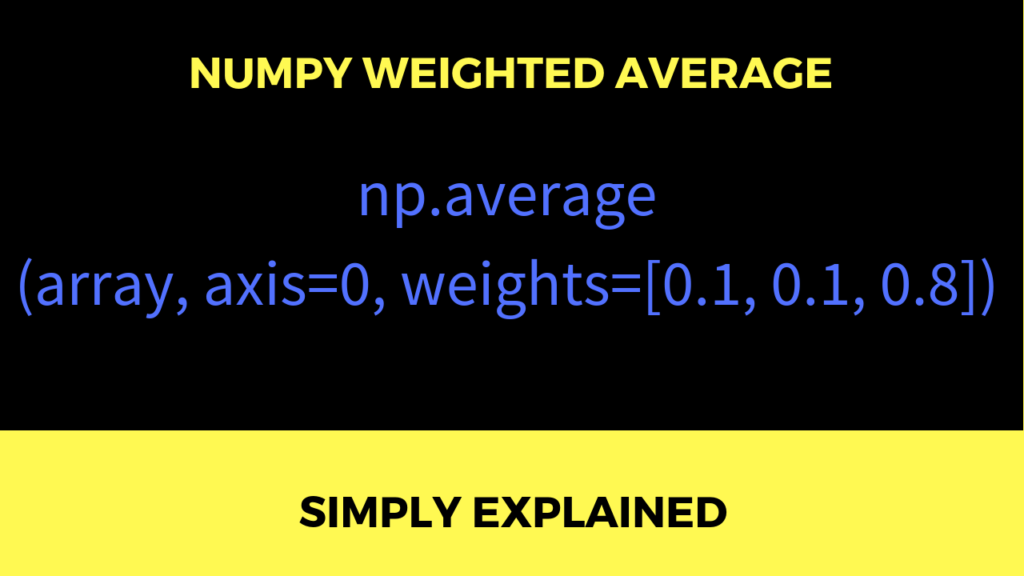# The Numpy Library

## Python’s Random Module – Everything You Need to Know to Get Started

Life is unpredictable. Sometimes good things happen out of the blue like you find \$100 on the floor. And sometimes bad things happen, like your flight being canceled because of bad weather. Most programming languages have a module to deal with randomness. Python is no exception coming with the module named random and in this …

## NumPy Datetime: How to Work with Dates and Times in Python?

In this article, we’ll be learning about something that is literally everywhere. Whatever corner you turn, whatever street you run down, you can’t get away from it. It is as ubiquitous as the physical space around us. Yes today, we’re talking about… TIME. More specifically, we’re talking about NumPy’s functions that represent dates and times. …

## Why does Python Look Great on Your Resume?

Nothing to add—let’s get started! 🐍 Python is the fastest-growing major programming language. A StackOverflow 2019 developer survey shows that Python is not only faster growing than C++, Java, JavaScript, and any other major programming language, it also comes with a happier community that loves the language most. Python is great for machine learning. Python comes with …

## [Collection] 10 Best NumPy Cheat Sheets Every Python Coder Must Own

Little time to learn NumPy? This article shows you the 10 most amazing NumPy cheat sheets. Download them, print them, and post them to your wall — and watch your data science skills grow! All NumPy cheat sheets in this article are 100% free. Here’s a quick summary if you don’t have time reading all …

## Why Should I Use NumPy?

NumPy is not the trendiest Python library in the world. There are libraries such as TensorFlow or Scikit-learn for machine learning. These libraries get all the attention these days. However, in this article, I want to highlight the importance of learning the basics first. You cannot learn TensorFlow before you’ve learned Python. Similarly, you cannot …

## A Python Implementation of NumPy Cumsum

You may know the np.cumsum() function from the NumPy library that calculates the cumulative sum of a given NumPy array. How can we implement this function in Python? Studying this will not only show you how the NumPy cumsum function works, but it’ll also make you a better coder because it contains many important concepts …

## How do You Slice and Index Multidimensional Arrays in Numpy?

Python’s library for data science, NumPy, allows you to slice multidimensional arrays easily. For multi-dimensional slices, you can use one-dimensional slicing for each axis separately. You define the slices for each axis, separated by a comma. Here are a few examples drawn from my comprehensive NumPy tutorial. Take your time to thoroughly understand each of …## How to Start Your Career as a Data Scientist with Python?

This guide is a conceptual view on how to start your career as a data scientist–and on which skills you should focus. Here are the three most important steps recommended in the video: Learn Python basics (link to free Python crash course on this blog). Learn NumPy (link to free NumPy tutorial on this blog). …## NumPy Tutorial – Everything You Need to Know to Get Started

This tutorial gives you a simple introduction to Python’s NumPy library. You don’t need any prerequisites to follow the tutorial. My goal was to give a practical and fun NumPy introduction for absolute beginners and with many examples. By reading through this tutorial, you will gain a basic understanding of the most important NumPy functionality. …## NumPy arange(): A Simple Illustrated Guide

The np.arange() function appears in 21% of the 35 million Github repositories that use the NumPy library! This illustrated tutorial shows you the ins and outs of the NumPy arange function. So let’s get started! What’s the NumPy arange function? The np.arange([start,] stop[, step]) function creates a new NumPy array with evenly-spaced integers between start …## How to Calculate the Weighted Average of a Numpy Array in Python?

NumPy’s average function computes the average of all numerical values in a NumPy array. When used without parameters, it simply calculates the numerical average of all values in the array, no matter the array’s dimensionality. For example, the expression np.average([[1,2],[2,3]]) results in the average value (1+2+2+3)/4 = 2.0. However, what if you want to calculate …